# Money management and its impact on trader’s results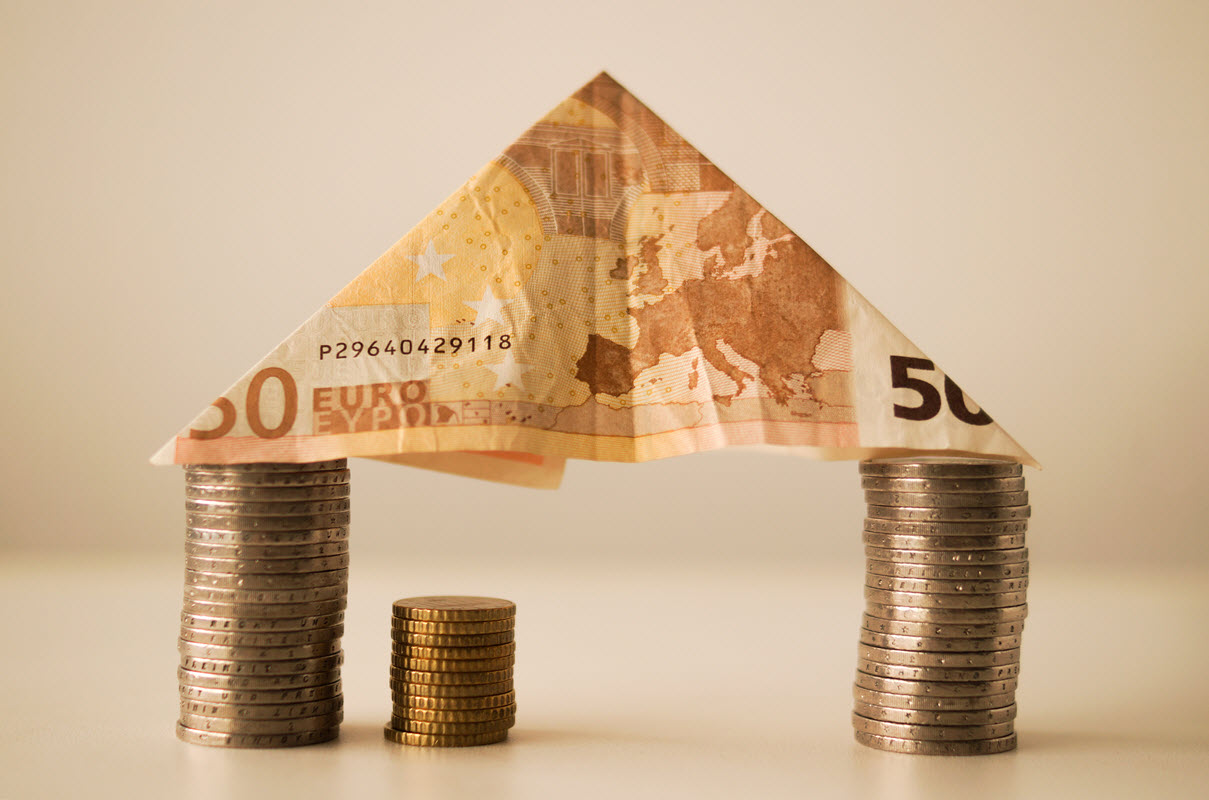###### byAlexander Mikhailenko

In the previous articles of the «Axioms of Trading» cycle, we discussed options for protecting trading capital from large drawdowns, as well as the advantages of applying these principles in situations when the market moves in the right direction during several trades in a row.

At the same time, all the examples were restricted by a small number of trades, whereas a trader gets the greatest benefit of risk and money management applications when using them during long periods of time.

In this article, we will take examples of the impact of money and risk management rules on long-term trade history when it comes to hundreds of trades.

## The impact of various money management styles on trade statistics

In Figure 1 you can see two yield curves that were modeled according to the following principles: the probability of profit in each trade is 42%, the risk-reward ratio is 1:2. Seed capital is \$1000.Fig. 1. The impact of various money-management methods on trade statistics. The probability of profit is 42%. Risk-reward ratio = 1:2. Green chart: the risk is determined as a share of capital (3%). Blue chart: the risk is fixed in the account currency (\$30).

This is a stress test so there are more losing trades than profitable ones. If a trader is often wrong, but he persistently follows money and risk management rules, then his trading account can still grow.

In this experiment, we’ll compare once again the impact of different options for determining the optimal position size on trade statistics. Blue chart. The risk per trade is fixed in the account currency. Green chart. The risk is determined as a share of capital (dynamic risk per trade). The vertical axis is capital, the horizontal axis is trades.

In the short-term, it is difficult to appreciate the advantages of using money and risk management rules. At the same time, if the account is increased by at least 50-70%, the gap between the results becomes significant.

### Don’t focus on making money; focus on protecting what you have.

Paul Tudor Jones — investor, hedge fund manager

If the risk per trade is fixed as a share of the trading capital, the yield curve becomes as close to the vertical as possible when the market goes in the desired direction. The effect of using this principle of determining the optimal position size is similar to using a compound interest when the profit is reinvested. In other words, if a trader does not withdraw profits from the trading account for a while, the use of money management rules contributes to the active growth of the trading account.

This is very clearly illustrated by the following fact. After the 300th trade with a fixed risk per trade in the account currency, the capital grows up to \$3430, whereas the application of the dynamic risk per trade gives a result equal to \$8400. The profit potential is three times higher.

I generated several similar experiments (Figures 2, 3 and 4). Conditions do not change, that is there are 300 trades, seed capital is \$1000, the probability of profit is 42% and the risk-reward ratio is 1:2.Fig. 2. The impact of various money-management methods on trade statistics. The probability of profit is 42%. Risk-reward ratio = 1:2. Green chart: the risk is determined as a share of capital (3%). Blue chart: the risk is fixed in the account currency (\$30).

When the account hardly grows the results of applying various methods of money management will be very similar. But if a trader determines the risk per trade as a share of capital, then in case of getting new profitable trades the effect of the financial result of these trading operations on the overall statistics becomes much more significant, that is the green chart grows much faster than the blue one.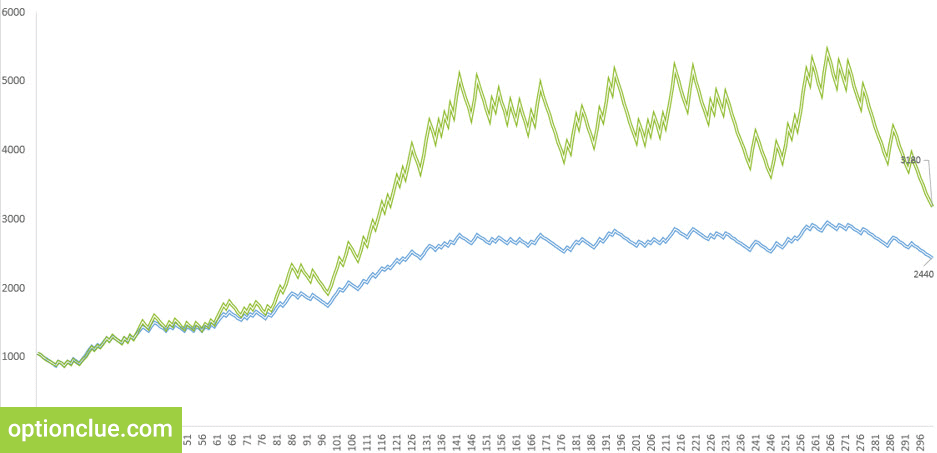Fig. 3. The impact of various money-management methods on trade statistics. The probability of profit is 42%. Risk-reward ratio = 1:2. Green chart: the risk is determined as a share of capital (3%). Blue chart: the risk is fixed in the account currency (\$30).

Until the capital has significantly increased, essential differences between various methods of money management are not observed. At the same time with the growth of the trading account yields get considerably different.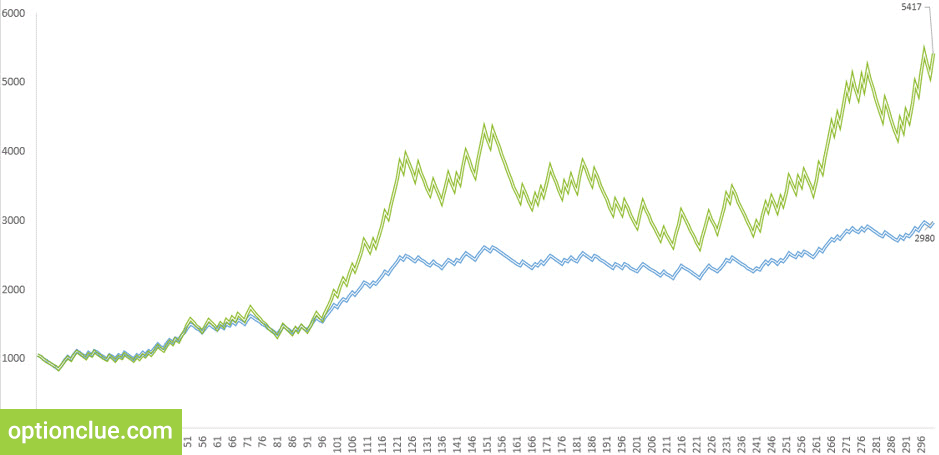Fig. 4. The impact of various money-management methods on trade statistics. The probability of profit is 42%. Risk-reward = 1:2. Green chart: the risk is determined as a share of capital (3%). Blue chart: the risk is fixed in the account currency (\$30).

In the following example (Figure 5) the risk management principles remain unchanged: the probability of profit, as before, is 42% and the risk-reward ratio is 1:2. Risk management did not change, but money management did and the risk per trade became higher: \$50 (blue chart) versus 5% of the capital (green chart).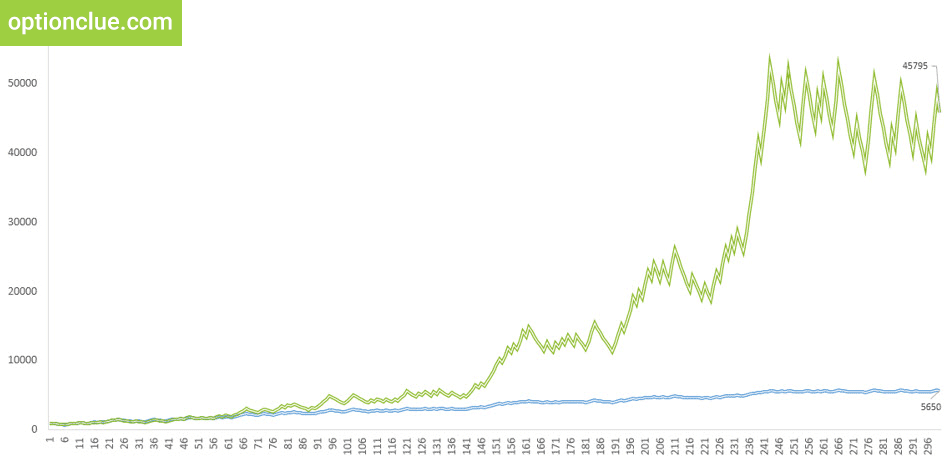Fig. 5. The impact of various money-management methods on trade statistics. The probability of profit is 42%. Risk-reward = 1:2. Green chart: the risk is determined as a share of capital (5%). Blue chart: the risk is fixed in the account currency (\$50).

In this example, the risk per trade is high (5% of the risk per trade is a highly aggressive trading), which affects the profit potential (+ 10% in each positive trade). Basically, the green chart reflects the returns with the reinvestment of the profit and blue one – without the reinvestment.

In this example, in the long-term, the difference between returns becomes much more significant. The lion’s share of trades is unprofitable and the trading account is growing due to the application of money and risk management rules. When using the fixed risk per trade in the account currency the capital grows from \$1000 to \$5,650. The application of the dynamic risk per trade generates the result equal to about \$45,000.

If the risk per trade is fixed as a share of the trading capital and the optimal position size is calculated according to the formula, then a positive effect of the profit reinvestment is formed and every cent is used as efficiently as possible.

Obviously, risking 5% of the capital per trade, as well as leaving all the profit on a trading account is not the best idea. The purpose of this illustration is to demonstrate the importance and advantages of using risk and money management rules in everyday trading practice.

In my opinion, the examples given above perfectly reflect the benefits that a trader gets when using risk management and money management. Every cent on the trading account is used efficiently, the capital grows much more actively and the effect of a compound interest is formed. If a trader withdraws profit from his trading account seldom, this approach will let use all available funds as efficiently as possible.

Using money and risk management rules you get a powerful competitive advantage over traders who do not use them. Even if you are wrong more often than you are right, your capital may increase. Let’s analyze another illustration of this thought.

## Efficiency of money management methods during drawdowns

350 trades were modeled for the following slides. The statistics is close to the one that can be obtained from real trading in the market. Vertical lines mark out trading periods. «Green» indicates a period of time when the probability of losing trade is 20% and profitable one is 80%. «Red» indicates periods of failure where the probability of losing trade is 80%.

Such illustration is worth modeling as it can clearly demonstrate what happens to a trading account if a trader trades the same concept and uses risk and money management rules during a long period of time.Fig. 6. The impact of various money-management methods on trade statistics. The probability of profit in the green range is 80% and in the red is 20%. Green chart: the risk is determined as a share of capital (3%). Blue chart: the risk is fixed in the account currency (\$30).

It is important to note that in order to create an additional effect of the stress test, we’ll keep in mind that profitable periods last only 30 trades and unsuccessful ones 50 trades. They alternate, that is 30 trades is a successful period, 50 ones is an unsuccessful period, 30 trades is again a successful period and 50 ones is again an unsuccessful period. And so on.

Let’s summarize the key conditions that are concluded in the model:

• The risk-reward ratio is 1:2
• Number of trades is 350
• Probability of profit in «green» period is 80%
• Probability of profit in «red» period is 20%
• Duration of «green» period is 30 trades (150 trades for each experiment)
• Duration of «red» period is 50 trades (200 trades for each experiment)

This is difficult time as most of the trades are unprofitable. How does this affect profitability? In illustrations 6 – 10 the yield curves are modeled according to the principles described above.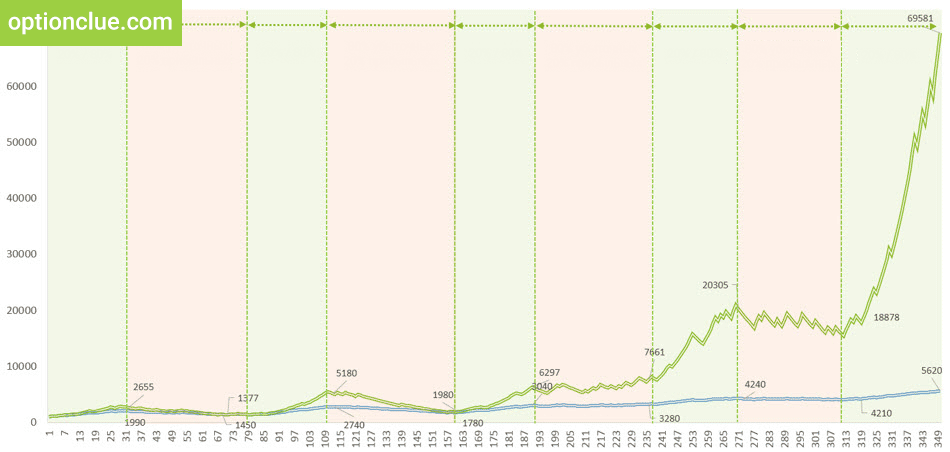Fig. 7. The impact of various money-management methods on trade statistics. The probability of profit in the green range is 80% and in the red is 20%. Green chart: the risk is determined as a share of capital (3%). Blue chart: the risk is fixed in the account currency (\$30).

The result is positive due to the application of money and risk management rules. The risk-reward ratio is 1:2 while the green chart shows much more attractive result in successful trading periods. The use of dynamic risk per trade allows you to reinvest every cent of profit and with a number of positive trades, a trader gets the most positive effect.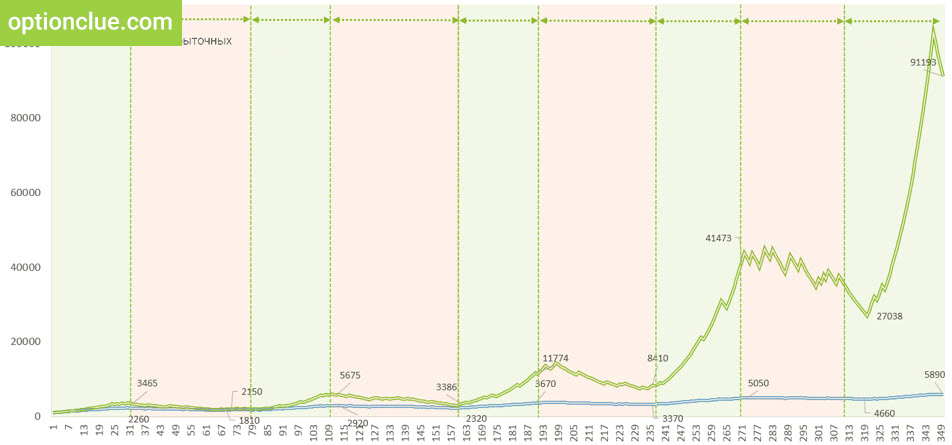Fig. 8. The impact of various money-management methods on trade statistics. The probability of profit in the green range is 80% and in the red is 20%. Green chart: the risk is determined as a share of capital (3%). Blue chart: the risk is fixed in the account currency (\$30).

If the risk per trade is fixed in the account currency, the capital grows slowly and decreases quickly (the drawdown as a percentage of capital in most cases will be much deeper, this issue is discussed in detail in the article «Basic methods of money management: their strengths and weaknesses»). In addition, if the risk is fixed in the account currency, a trader can destroy the trading capital in case of several losing trades in a row.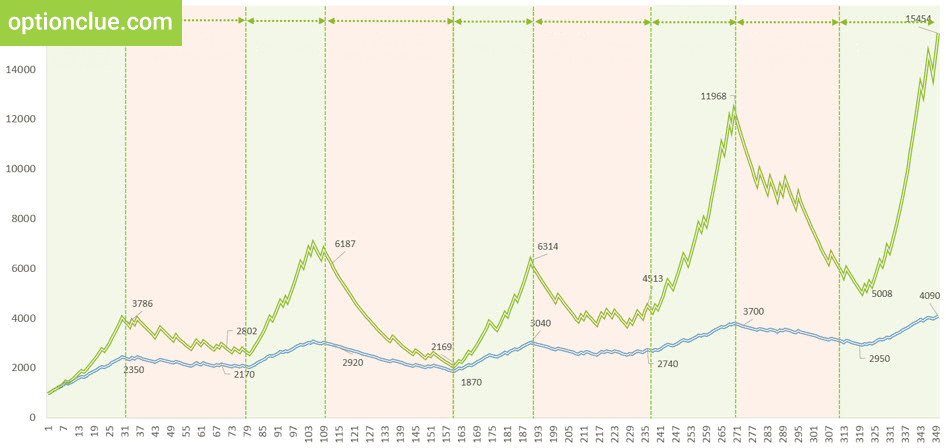Fig. 9. The impact of various money-management methods on trade statistics. The probability of profit in the green range is 80% and in the red is 20%. Green chart: the risk is determined as a share of capital (3%). Blue chart: the risk is fixed in the account currency (\$30).

These illustrations perfectly emphasize the advantages that a trader gets when using money and risk management rules. When the market goes against a trader during several trades, he gets the opportunity to save his capital in order to trade further. When the market goes in the desired direction a trader maximizes the profit. If the risk per trade is determined as a share of capital, the yield curve strives to vertical. Otherwise, it does not reach the vertical if this principle is not applied.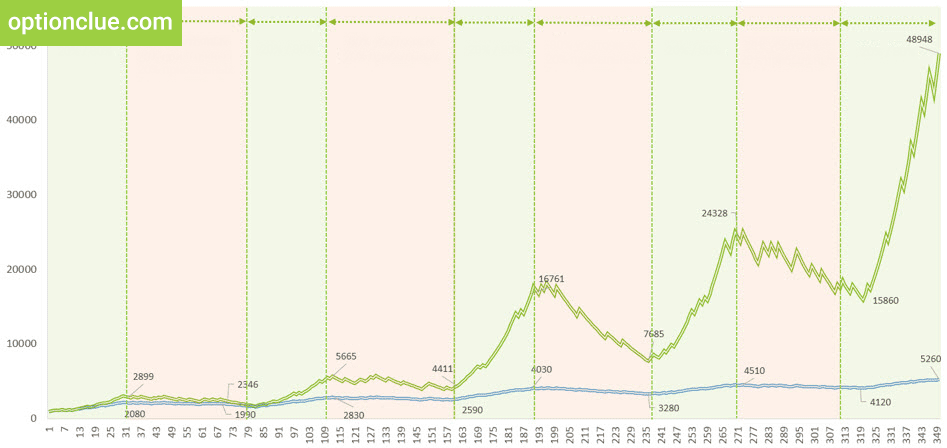Fig. 10. The impact of various money-management methods on trade statistics. The probability of profit in the green range is 80% and in the red is 20%. Green chart: the risk is determined as a share of capital (3%). Blue chart: the risk is fixed in the account currency (\$30).

In the examples described above profit is implied not to be withdrawn at all. It is obvious that in most cases it makes no sense to accumulate profit. But if the account grows and the financial result remains for some time within the trading account, each dollar received is reinvested and used as efficiently as possible.

A trader gets undeniable advantages both in the «green» zone when the market moves more often in the desired direction, and in the «red» zone when negative trades occur more often than positive ones.

You can see that drawdowns become more significant in monetary terms when applying dynamic risk per trade. Reinvesting every cent a trader gets the opportunity to maximize profit, but he risks this capital. This decision must be conscious.

### Conclusions on the importance of money management rules in trading

When trading in the financial markets a trader always has a choice. Whether to use every cent as efficiently as possible or not? Whether to lose a trading account or not when the market moves in the unfavorable direction ten or fifteen trades in a row?

The answers to these questions are directly related to the use of money and risk management rules, in particular, the application of dynamic risk per trade. This is the principle that smoothes the trading account drawdowns and creates the maximum positive effect when the market goes in the right direction.

Most traders lose money in the market since they do not use risk and money management rules in their trading practices. Therefore, if these principles become an integral part of your trading strategy, you will gain a powerful competitive advantage in this kind of business.